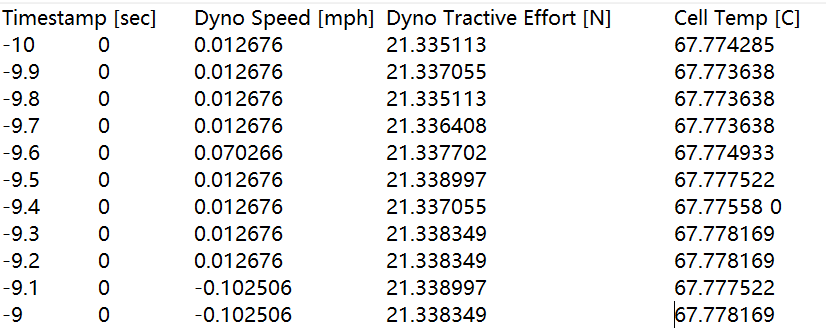# MATLAB数据文件处理（txt，mat，excel等）

## txt文件

txt数据采集得到的示例样式如下：1、第一行为数据的名称
2、从第二行开始为每个变量对应的时间序列采集数据值
3、数据的名称和数据的数值之间均通过 tab 分割

#### 处理方法

1. Data = regexp(text,’\r\n|\n’,‘split’)’;
解释： 将每一行的数据分割为每一个cell类型，整个text文件的每一行均对应Data 这个cell元胞数组的每一个元素
2. VarNames = regexp(Data{1},’\t’,‘split’);
解释：对文本的第一行的数据名称提取
3. VarNames = regexprep(VarNames, ‘^\s*|\s*$’, ‘’); 解释：对数据名称最前面的空白或者最后面的空白去除，例如名称为 ‘ __ var __’ 转换为‘var’ 4. Data_instant = str2num(Data{i}); 解释：对每一行的数据转换为数值 function MatData = readTextFile(FilePath) fid = fopen(FilePath); text = fscanf(fid, '%c'); fclose(fid); Data = regexp(text,'\r\n|\n','split')'; while isempty(Data{end}) Data(end) = []; end VarNames = regexp(Data{1},'\t','split'); VarNames = regexprep(VarNames, '^\s*|\s*$', '');
Values = zeros((length(Data)-1), length(VarNames));
length_VarNames = length(VarNames);
IdxToDelete = [];
IdxEmptyLine = [];
length_data = length(Data);
for i=2:1:length_data
Data_instant = str2num(Data{i});
if length(Data_instant) == length_VarNames
Values(i-1,:) = Data_instant;
elseif isempty(Data_instant)
IdxEmptyLine = [IdxEmptyLine, i-1];
else
IdxToDelete = [IdxToDelete, i-1];
end
end
if length(IdxToDelete)>1000
msgbox(['数据中有太多不符合规范，请使用正确的格式重试'],'规范不符')
end
Values(IdxEmptyLine, :) = [];
Values(IdxToDelete, :) = [];
if ~isempty(IdxToDelete)
msgbox(['Line(s) ', num2str(IdxToDelete),' are delete.'],'Unexpected Data')
end
MatData.Values = Values;
MatData.VarNames = cell(1, length(VarNames));


function [ VarName, Unit ] = separateNameAndUnitForRawData( VarName )
if ~ischar( VarName )
Unit = [  ];
return ;
end
Unit = converCell2String( regexp( VarName, '$.*$', 'match' ) );
Unit = regexprep( Unit, '$|$', '' );
end被折叠的  条评论 为什么被折叠?到【灌水乐园】发言AutoMarie

¥2 ¥4 ¥6 ¥10 ¥20余额支付 (余额：-- )扫码支付获取中扫码支付点击重新获取扫码支付1.余额是钱包充值的虚拟货币，按照1:1的比例进行支付金额的抵扣。
2.余额无法直接购买下载，可以购买VIP、C币套餐、付费专栏及课程。余额充值